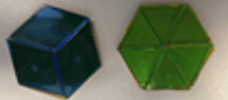Teachers and students need to communicate using how many groups/sets/lengths are in a given amount. Students can apply their understanding of whole number division as they build understanding of division with fractions.

Students may struggle to visualize division of fractions. Asking students, ‘what is one-third divided by one-half’ doesn’t connect to any particular visual. Instead, ask a question that connects to how students visualized division of whole numbers. 8 ÷ 4; How many groups of 4 are in 8? Using only one fraction is also a way to bridge into division of fractions. 4 ÷ 1/2; How many halves are in 4?Pattern blocks can be used to show division of more complicated fractions.How many one-sixths are in one-third?